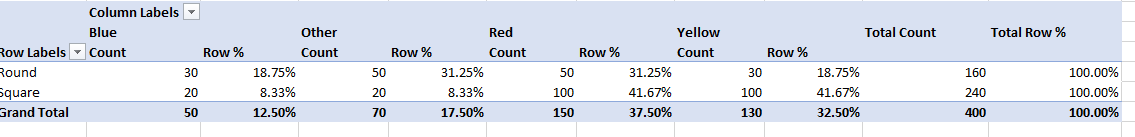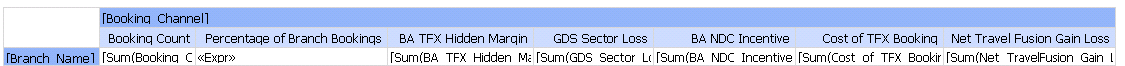# SSRS SUM(IIF()) on a matrix.

• david_h_edmonds

Ten Centuries

Points: 1232

Hi, apologies if I am asking a question that has been asked many times but I can't find the answer that I need.

I have a matrix in ssrs and I am wanting to add a column into it that shows a value as a perentage of the row total as you can do in excel as follows:I have tried sum(iff()
I have tried sum/sum
I have tried helper fields and I still can't get it going.
Does anyone have a way to explain this for me please?
Expr for the field is:
=sum(Fields!Booking_Count.Value,"matrix1_Booking_Channel")/sum(Fields!Booking_Count.Value)
My matrix is as follows:Cheers,

Dave

• Sue_H

SSC Guru

Points: 89874

david_h_edmonds - Thursday, March 28, 2019 7:53 AM

Hi, apologies if I am asking a question that has been asked many times but I can't find the answer that I need.

I have a matrix in ssrs and I am wanting to add a column into it that shows a value as a perentage of the row total as you can do in excel as follows:I have tried sum(iff()
I have tried sum/sum
I have tried helper fields and I still can't get it going.
Does anyone have a way to explain this for me please?
Expr for the field is:
=sum(Fields!Booking_Count.Value,"matrix1_Booking_Channel")/sum(Fields!Booking_Count.Value)
My matrix is as follows:Cheers,

Dave

Percent of the row total would just be the count/total count in the row * 100. I can't tell where the total count is on your matrix, if there are multiple rows with a totals row, grouped by channel, branch or however it's set up.
In your first example, the expression for the Blue percent of row would be the blue count / total count for all the colors in the row * 100.
`=Sum(Fields!BlueCount.Value)/Sum(Fields!BlueCount.Value + Fields!OtherCount.Value + Fields!RedCount.Value + Fields!YellowCount.Value)*100`

Sue

• david_h_edmonds

Ten Centuries

Points: 1232

Sue_H - Thursday, March 28, 2019 7:14 PM

david_h_edmonds - Thursday, March 28, 2019 7:53 AM

Hi, apologies if I am asking a question that has been asked many times but I can't find the answer that I need.

I have a matrix in ssrs and I am wanting to add a column into it that shows a value as a perentage of the row total as you can do in excel as follows:I have tried sum(iff()
I have tried sum/sum
I have tried helper fields and I still can't get it going.
Does anyone have a way to explain this for me please?
Expr for the field is:
=sum(Fields!Booking_Count.Value,"matrix1_Booking_Channel")/sum(Fields!Booking_Count.Value)
My matrix is as follows:Cheers,

Dave

Percent of the row total would just be the count/total count in the row * 100. I can't tell where the total count is on your matrix, if there are multiple rows with a totals row, grouped by channel, branch or however it's set up.
In your first example, the expression for the Blue percent of row would be the blue count / total count for all the colors in the row * 100.
`=Sum(Fields!BlueCount.Value)/Sum(Fields!BlueCount.Value + Fields!OtherCount.Value + Fields!RedCount.Value + Fields!YellowCount.Value)*100`

Sue

Hi Sue, thanks for the reply. I am after a dynamic expression something like:

`=Sum(current column group member)/Sum(all column group members)*100 `

Is this even possible?

Cheers

Dave

• David Burrows

SSC Guru

Points: 64444

Think you want something like this
`=(Sum(Fields!Booking_Count.Value)/ReportItems!Textbox1.Value)*100`

Replace Textbox1 with the actual name of the Total Count textbox

Far away is close at hand in the images of elsewhere.
Anon.

• david_h_edmonds

Ten Centuries

Points: 1232

David Burrows - Friday, March 29, 2019 7:15 AM

Think you want something like this
`=(Fields!Booking_Count.Value/ReportItems!Textbox1.Value)*100`

Replace Textbox1 with the actual name of the Total Count textbox

Ok this could work but there is 1 more complication, there is a total for each row of the matrix so I would need a total for each member of the row group and then lookup up the correct total based on the row group member.

Revising what I put earlier, that alters to:

`=Sum(current column group member and row group member)/Sum(all column group members for current row group member)*100 `
How could I achieve this?
Or am I missing the point? I am honestly so confused by this, Excel does it in 2 clicks and this seems so complicated.

Many thanks,
Dave

• David Burrows

SSC Guru

Points: 64444

Sorry missed the Sum

`=(Sum(Fields!Booking_Count.Value)/ReportItems!Textbox1.Value)*100`

*Edited my original post as well

Far away is close at hand in the images of elsewhere.
Anon.

Viewing 6 posts - 1 through 6 (of 6 total)